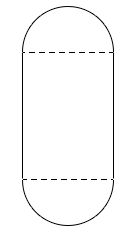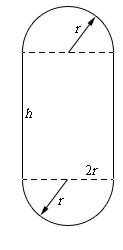Paul's Online Notes
Home / Calculus I / Applications of Derivatives / More Optimization Problems
Show Mobile Notice Show All Notes Hide All Notes
Mobile Notice
You appear to be on a device with a "narrow" screen width (i.e. you are probably on a mobile phone). Due to the nature of the mathematics on this site it is best views in landscape mode. If your device is not in landscape mode many of the equations will run off the side of your device (should be able to scroll to see them) and some of the menu items will be cut off due to the narrow screen width.

### Section 4.9 : More Optimization

1. We want to construct a window whose middle is a rectangle and the top and bottom of the window are semi-circles. If we have 50 meters of framing material what are the dimensions of the window that will let in the most light?Show All Steps Hide All Steps

Start SolutionShow Step 2

Next, we need to set up the constraint and equation that we are being asked to optimize.

We are told that we have 50 meters of framing material (i.e. the perimeter of the window) and so that will be the constraint for this problem.

$50 = 2h + 2\left( {\pi r} \right) = 2h + 2\pi r$

We are being asked to maximize the amount of light being let in and that is simply the enclosed area or,

$A = h\left( {2r} \right) + 2\left( {{\frac{1}{2}}\pi {r^2}} \right) = 2hr + \pi {r^2}$

With both of these equations we were a little careful with the last term. In each case we needed either the perimeter or area of each semicircle and there were two of them. The end result of course is the equation of the perimeter/area of a whole circle, but we really should be careful setting these equations up and note just where everything is coming from.

Show Step 3

Now, let’s solve the constraint for $$h$$.

$h = 25 - \pi r$

Plugging this into the area function gives,

$A\left( r \right) = 2\left( {25 - \pi r} \right)r + \pi {r^2} = 50r - \pi {r^2}$ Show Step 4

Finding the critical point(s) for this shouldn’t be too difficult at this point. Here is the derivative.

$A'\left( r \right) = 50 - 2\pi r$

From this it looks like we get a single critical points : $$r = \frac{25}{\pi} = 7.9577$$.

Show Step 5

The second derivative of the volume function is,

$A''\left( r \right) = - 2\pi$

From this we can see that the second derivative is always negative. Therefore $$A\left( r \right)$$ will always be concave down and so the single critical point we got in Step 4 must be a relative maximum and hence must be the value that allows in the maximum amount of light.

Show Step 6

Now, let’s finish the problem by getting the radius of the semicircles.

$h = 25 - \pi \left( \frac{25}{\pi} \right) = 0$

Okay, what this means is that in fact the most light will come from not even having a rectangle between the semicircles and just having a circular window of radius $$r = \frac{25}{\pi}$$.